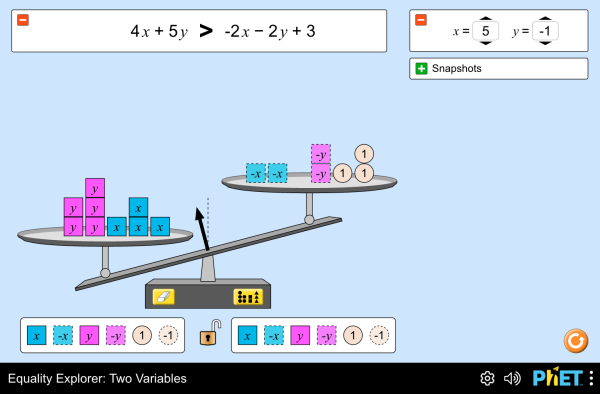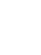# Equality Explorer: Two Variables

Aceste simulări nu au fost încă traduse în limba română . Puteți accesa simularea, din meniul de mai jos, în limba engleză.DescarcăInclude Închide Include o copie functională a simulării Folosiţi acest cod sursă pentru a include o simulare. Puteţi mofifica dimensiunile prin modificarea parametrilor "width" şi "height". Include o imagine care lansează simularea
Rulează
Folosiţi acest cod sursă pentru afişarea "Rulează" Equations Inequalities System of Equations PhET is supported byand educators like you.

### Subiecte

• Equations
• Inequalities
• System of Equations

### Descriere

Explore what it means for a mathematical statement to be balanced or unbalanced by interacting with integers and variables on a balance. Find multiple ways to balance x and y to build a system of equations.

### Studii de caz

• Solve and manipulate algebraic equations by substituting different values for a variable
• Use a balance model to solve an equation for an unknown, and justify your strategies for solving
• Build a system of equations
• Determine the solution set of a system of equations

### Standards Alignment

#### Common Core - Math

6.EE.B.5
Understand solving an equation or inequality as a process of answering a question: which values from a specified set, if any, make the equation or inequality true? Use substitution to determine whether a given number in a specified set makes an equation or inequality true.
6.EE.B.6
Use variables to represent numbers and write expressions when solving a real-world or mathematical problem; understand that a variable can represent an unknown number, or, depending on the purpose at hand, any number in a specified set.
7.EE.B.3
Solve multi-step real-life and mathematical problems posed with positive and negative rational numbers in any form (whole numbers, fractions, and decimals), using tools strategically. Apply properties of operations to calculate with numbers in any form; convert between forms as appropriate; and assess the reasonableness of answers using mental computation and estimation strategies. For example: If a woman making \$25 an hour gets a 10% raise, she will make an additional 1/10 of her salary an hour, or \$2.50, for a new salary of \$27.50. If you want to place a towel bar 9 3/4 inches long in the center of a door that is 27 1/2 inches wide, you will need to place the bar about 9 inches from each edge; this estimate can be used as a check on the exact computation.
7.EE.B.4
Use variables to represent quantities in a real-world or mathematical problem, and construct simple equations and inequalities to solve problems by reasoning about the quantities.
8.EE.C.7
Solve linear equations in one variable.
8.EE.C.7a
Give examples of linear equations in one variable with one solution, infinitely many solutions, or no solutions. Show which of these possibilities is the case by successively transforming the given equation into simpler forms, until an equivalent equation of the form x = a, a = a, or a = b results (where a and b are different numbers).
8.EE.C.8
Analyze and solve pairs of simultaneous linear equations.
HSA-CED.A.1
Create equations and inequalities in one variable and use them to solve problems. Include equations arising from linear and quadratic functions, and simple rational and exponential functions.
HSA-CED.A.3
Represent constraints by equations or inequalities, and by systems of equations and/or inequalities, and interpret solutions as viable or nonviable options in a modeling context. For example, represent inequalities describing nutritional and cost constraints on combinations of different foods.
HSA-REI.A.1
Explain each step in solving a simple equation as following from the equality of numbers asserted at the previous step, starting from the assumption that the original equation has a solution. Construct a viable argument to justify a solution method.
HSA-REI.B.3
Solve linear equations and inequalities in one variable, including equations with coefficients represented by letters.
Versiune 1.0.12

### Sfaturi pentru profesoriGhidul profesorului conține un sumar al elementelor de control ale simulării, modelul ideal, perspective asupra gândirii elevilor ( PDF ).

### Activități ale profesorilor

Middle School Math Sim AlignmentAmanda McGarry MS Other Mathematics
SECUNDARIA: Alineación PhET con programas de la SEP México (2011 y 2017)Diana López MS
HS
Other Chemistry
Physics
Biology
Mathematics
PREPARATORIA: Alineación de PhET con programas de la DGB México (2017)Diana López HS
UG-Intro
Other Mathematics
Chemistry
Physics
Limba Downloador RunTips
Albanian All shqipHulumtues barazishë: Dy ndryshore
Arabic All العربيةمستكشف المساواة: متغيرين
Basque All EuskaraBerdintasun-esploratzailea: bi aldagai
Bosnian All BosanskiIstraživač jednačina: Dvije promjenljive
Brazilian Portuguese All português (Brasil)Explorador da Igualdade: Duas Variáveis
Chinese - Simplified All 中文 (中国)等式探索:两个变量
Chinese - Traditional All 中文 (台灣)Equality Explorer: Two Variables_探索等式：兩個變數
Danish All DanskLigevægts-visning: To variabler
Dutch All NederlandsOntdek gelijkheid: twee veranderlijken
French All françaisExplorateur d'équation : Deux variables
German All DeutschGleichheit schaffen: Zwei Variablen
Greek All ΕλληνικάΕξερευνητής εξισώσεων: δυο μεταβλητές
Gujarati All Gujaratiસમીકરણ સમાનતા શોધક: બે ચલ- Pankajsid34
Hindi All हिंदीसमानता का पता करें : दो चरांको के
Hungarian All magyarEgyenlőségvizsgálat: két változó
Italian All italianoEsplora le equazioni a due variabili
Japanese All 日本語つり合いを作ろう: 2つの文字を用いて
Kazakh All KazakhЕкі айнымалы теңдік
Korean All 한국어등식 연습하기: 2변수
Latvian All LatviešuVienādību pētīšana: Divi mainīgie
Mongolian All Монгол (Монгол)Тэнцэтгэл Судлах: Хоёр Хувьсагч
Persian All فارسیکاوشگر برابری: دو متغیر
Polish All polskiPoznajemy równania - dwie niewiadome
Portuguese All portuguêsExplorador de Igualdades. Duas Variáveis
Russian All русскийРавенства с двумя переменными
Serbian All СрпскиИстраживач једначина: Две променљиве
Slovak All SlovenčinaSkúmanie rovnosti: Dve premenné
Spanish All españolExplorador de Igualdades: Dos variablesSpanish (Mexico) All español (México)Explorador de Igualdades: Dos variablesSpanish (Peru) All español (Perú)Explorador de Igualdad: Dos Variables
Swedish All svenskaUtforska likheter: Två variabler
Turkish All TürkçeEşitlik Gezgini: İki Değişkenli
Ukrainian All українськаДослідник рівностей. Дві змінніVietnamese All Tiếng ViệtĐẳng thức: 2 biến

HTML5 sims can run on iPads and Chromebooks, as well as PC, Mac, and Linux systems.

iOS 12+ Safari

Android:
Not officially supported. If you are using the HTML5 sims on Android, we recommend using the latest version of Google Chrome.

Chromebook:
The HTML5 and Flash PhET sims are supported on all Chromebooks.
Chromebook compatible sims

Windows Systems:

Macintosh Systems:

Linux Systems:

Echipa de proiectare Alţi colaboratori Mulţumiri
• Chris Malley (developer)
• Diana López Tavares
• Ariel Paul
• Kathy Perkins
• Argenta Price
• David Webb
• Mariah Hermsmeyer (graphic arts)
• Cheryl McCutchan (graphic arts)
• almond-0.2.9.js
• base64-js-1.2.0.js
• easing-equations-r12
• FileSaver-b8054a2.js
• font-awesome-4.5.0
• game-up-camera-1.0.0.js
• he-1.1.1.js
• himalaya-0.2.7.js
• jama-1.0.2
• jquery-2.1.0.js
• lodash-4.17.4.js
• pegjs-0.7.0.js
• seedrandom-2.4.2.js
• text-2.0.12.js
• TextEncoderLite-3c9f6f0.js
• Tween-r12.js